# AVL Tree Deletion | Complete Guide to AVL Tree Deletion

17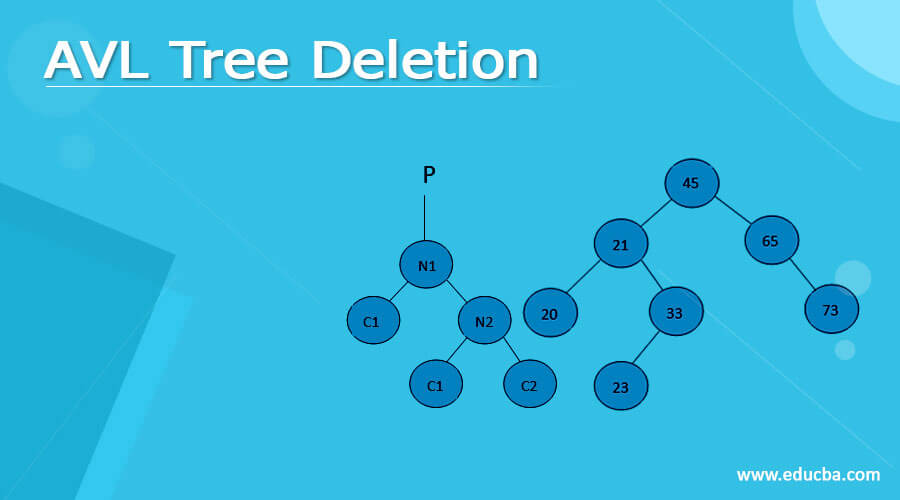## Definition of AVL Tree

AVL tree is also known as self-balancing binary search tree (BST) because each node in this tree has extra information about the node or the position of the node known as the balance factor. The balance factor contains -1, 0 or +1.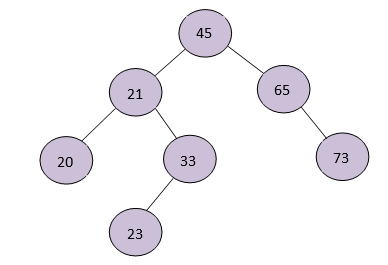### Deletion Operation in AVL Tree

When we delete an element in the AVL tree, this disturbs the balance factor of the whole tree for which the tree needs to be balanced again. To rebalance it, rotations are performed on it namely Left Rotation and Right rotation.

#### Left Rotation

1. Let’s assume the below AVL tree for left rotation.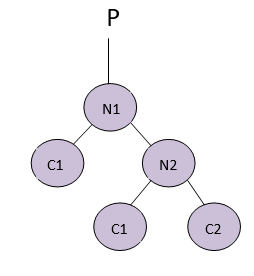2. If N2 has a left subtree, then assign N1 as the parent of the left subtree of N2.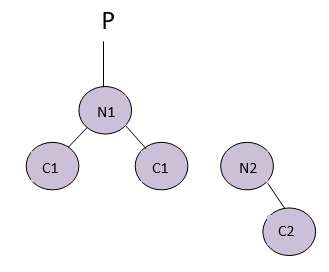• If N1 doesn’t have a parent, make N2 the parent of N1, which is the root of the tree.
• If N1 is the left child of any parent, then make N2 the left child of that parent.
• If the above two conditions don’t work, then make N2 the right child of the parent.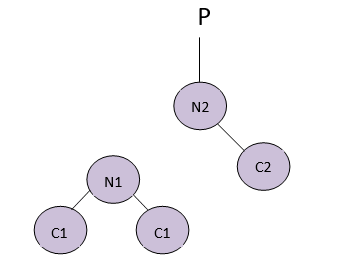This is the final balanced tree when we perform Left rotation on it. Right rotation is also done similarly to the AVL tree.

###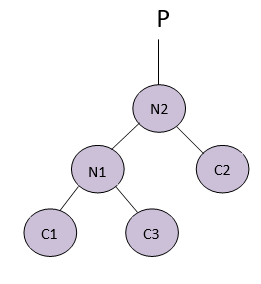Algorithm to delete an element in AVL tree

A node should always be deleted as a leaf node. When a node is deleted the balance factors of the tree get disturbed and there is a need to rebalance the tree to get the correct balance factors.

1. Find the node to be deleted using a recursion algorithm.
2. There are three conditions to delete a node
• If the node which is to be deleted is a leaf node, then that node is removed directly.
• If the node contains one child then swap them and then delete the leaf node which contains the key element.
• If the node contains two children, then find the inorder successor of that node which is to be deleted, that is, the value which is less than the key will be at the right subtree.
1. Substitute the node which is to be deleted with the before node.
2. Remove the substituted leaf node.
3. Balance the tree using any suitable rotation techniques.

Source Code

```# AVL tree implementation in Python import sys # Create a tree node class TreeNode(object): def __init__(self, key): self.key = key self.left = None self.right = None self.height = 1 class AVLTree(object): # Function to insert a node def insert_node(self, root, key): # Find the correct location and insert the node if not root: return TreeNode(key) elif key < root.key: root.left = self.insert_node(root.left, key) else: root.right = self.insert_node(root.right, key) root.height = 1 + max(self.getHeight(root.left), self.getHeight(root.right)) # Update the balance factor and balance the tree balanceFactor = self.getBalance(root) if balanceFactor > 1: if key < root.left.key: return self.rightRotate(root) else: root.left = self.leftRotate(root.left) return self.rightRotate(root) if balanceFactor < -1: if key > root.right.key: return self.leftRotate(root) else: root.right = self.rightRotate(root.right) return self.leftRotate(root) return root # Function to delete a node def delete_node(self, root, key): # Find the node to be deleted and remove it if not root: return root elif key < root.key: root.left = self.delete_node(root.left, key) elif key > root.key: root.right = self.delete_node(root.right, key) else: if root.left is None: temp = root.right root = None return temp elif root.right is None: temp = root.left root = None return temp temp = self.getMinValueNode(root.right) root.key = temp.key root.right = self.delete_node(root.right, temp.key) if root is None: return root # Update the balance factor of nodes root.height = 1 + max(self.getHeight(root.left), self.getHeight(root.right)) balanceFactor = self.getBalance(root) # Balance the tree if balanceFactor > 1: if self.getBalance(root.left) >= 0: return self.rightRotate(root) else: root.left = self.leftRotate(root.left) return self.rightRotate(root) if balanceFactor < -1: if self.getBalance(root.right) <= 0: return self.leftRotate(root) else: root.right = self.rightRotate(root.right) return self.leftRotate(root) return root # Function to perform left rotation def leftRotate(self, z): y = z.right T2 = y.left y.left = z z.right = T2 z.height = 1 + max(self.getHeight(z.left), self.getHeight(z.right)) y.height = 1 + max(self.getHeight(y.left), self.getHeight(y.right)) return y # Function to perform right rotation def rightRotate(self, z): y = z.left T3 = y.right y.right = z z.left = T3 z.height = 1 + max(self.getHeight(z.left), self.getHeight(z.right)) y.height = 1 + max(self.getHeight(y.left), self.getHeight(y.right)) return y # Get the height of the node def getHeight(self, root): if not root: return 0 return root.height # Get balance factore of the node def getBalance(self, root): if not root: return 0 return self.getHeight(root.left) - self.getHeight(root.right) def getMinValueNode(self, root): if root is None or root.left is None: return root return self.getMinValueNode(root.left) def preOrder(self, root): if not root: return print("{0} ".format(root.key), end="") self.preOrder(root.left) self.preOrder(root.right) # Print the tree def printHelper(self, currPtr, indent, last): if currPtr != None: sys.stdout.write(indent) if last: sys.stdout.write("R----") indent += " " else: sys.stdout.write("L----") indent += "| " print(currPtr.key) self.printHelper(currPtr.left, indent, False) self.printHelper(currPtr.right, indent, True) myTree = AVLTree() root = None nums = [33, 13, 52, 9, 21, 61, 8, 11] for num in nums: root = myTree.insert_node(root, num) myTree.printHelper(root, "", True) key = 13 root = myTree.delete_node(root, key) print("After Deletion: ") myTree.printHelper(root, "", True)```

Output:Time Complexity of Deletion in AVL tree

The time complexity of the AVL tree will be the same as Binary Search Tree. Getting the balance factor by performing various rotating operations and updating the height of the balance tree takes constant time. So, the time complexity will be similar to the time complexity of the Binary search tree which is O(h), where h is the height of the tree. Since the height of the AVL tree is log, the time complexity of Deleting an element in the AVL tree will be O(logn).

### Conclusion

• AVL tree is also known as self-balancing binary search tree(BST) because each node in this tree has extra information about the node or the position of the node known as the balance factor.
• We delete an element only in the leaf node in an AVL tree, if the key is not present in the leaf node we perform in order operations to bring it to the leaf node, then we delete the element.
• When we delete an element in the AVL tree, this disturbs the balance factor of the whole tree for which the tree needs to be balanced again.
• The time complexity of the Deletion operation of an AVL tree is O(logn)

### Recommended Articles

This is a guide to AVL Tree Deletion. Here we discuss the definition, Deletion Operation in AVL Tree, Algorithm to delete an element in the AVL tree. You may also have a look at the following articles to learn more –

The post AVL Tree Deletion appeared first on EDUCBA.